### Home > CALC > Chapter 3 > Lesson 3.4.2 > Problem3-168

3-168.
1. Evaluate each limit. If the limit does not exist, say so but also state if y is approaching positive or negative infinity. Homework Help ✎

1.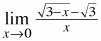2.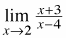3.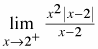4.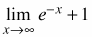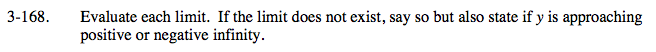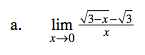This is Ana's definition of the derivative:

$f'(a)=\lim_{x\rightarrow a}\frac{f(x)-f(a)}{x-a}$

$\text{That means }f(x)=\sqrt{3-x}\text{ and }a=0.$

Find f '(x) and then the limit will equal f '(0).

Or you could multiply the numerator and denominator by the conjugate of the numerator.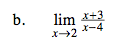Evaluate. The denominator will not equal zero so the limit and the actual value agree at x = 2.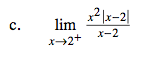Rewrite the absolute value as a piecewise function but, since x → 2+, only consider the piece in which x > 2.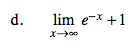Think about what the graph of y = ex looks like. What does it look like all the way to the right, as x → ∞? How does the +1 affect the graph?